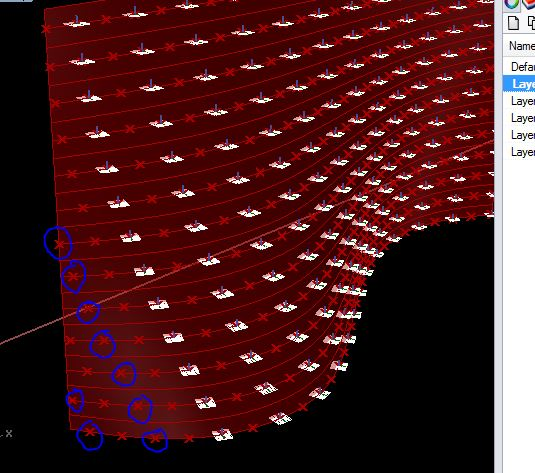# c#_DataTree_alternate selection of Objects

Hello,
I have divided the curves by length factor. I want to select the output points alternatively. I am able to sort them alternatively using DataTree. Since all the branch item counts are not same, last few points I am not able to select.
Any help in this regard would be great!

ps.1) I am pretty new to c# and trying out data tree in c# for the first time. I would like to know more about it, if there is any material out on this please let me know.

1. Attached image, script and GH file with internalised geometry

DataTree < Point3d > pD1 = new DataTree();
DataTree < Point3d > fD1 = new DataTree();

// bC1 is list of curves

``````for(int k = 0; k < bC1.Count; k++)
{
Point3d[] p1;
bC1[k].DivideByLength(0.5, true, out p1);
GH_Path gP1 = new GH_Path(k);
}
for(int l = 0; l < pD1.BranchCount; l++)
{
for(int m = 0;m < pD1.Branch(3).Count;m += 2)
{
GH_Path gF1 = new GH_Path(l);
}
}

B = pD1;
C = fD1;
``````05_Brick Wall.gh (23.3 KB)

for(int l = 0; l < pD1.BranchCount; l++)
{
GH_Path gF1 = new GH_Path(l);
for(int m = 0;m < pD1.Branch(gF1).Count;m += 2)
{